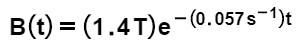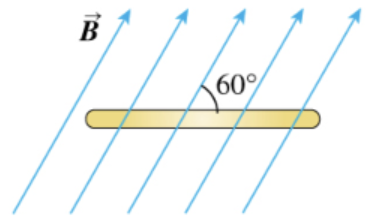# Problem: A flat, circular, steel loop of radius 75 cm is at rest in a uniform magnetic field, as shown in an edge-on view in the figure. The field is changing with time, according toε(t) = 0.122e-(0.057)tWhen is the induced emf equal to 1/18 of its initial value?

###### FREE Expert Solution

Comparing with ε(t) = ε0e-ct

ε0 = 0.122

(1/18)ε0 = 0.122/18###### Problem Details

A flat, circular, steel loop of radius 75 cm is at rest in a uniform magnetic field, as shown in an edge-on view in the figure. The field is changing with time, according toε(t) = 0.122e-(0.057)tWhen is the induced emf equal to 1/18 of its initial value?